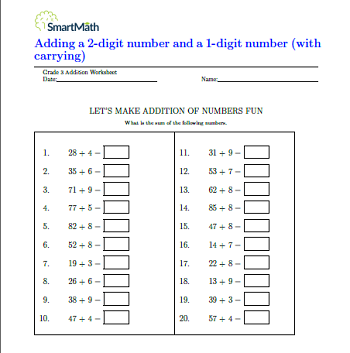# Adding a 2-digit number and a 1-digit number (with carrying)

Adding a 2-digit number and a 1-digit number (with
carrying)

LET’S MAKE ADDITION OF NUMBERS FUN

What is the sum of the following numbers.

Scroll to Top